# Multi Class Text Classification with Keras and LSTM

In this tutorial, we will build a text classification with Keras and LSTM to predict the category of the BBC News articles.

## LSTM (Long Short Term Memory)

LSTM was designed to overcome the problems of simple Recurrent Network (RNN) by allowing the network to store data in a sort of memory that it can access at a later times. LSTM is a special type of Recurrent Neural Network (RNN) that can learn long term patterns.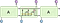Source: https://colah.github.io/posts/2015-08-Understanding-LSTMs/

The key to LSTMs is the cell state, the horizontal line running through the top of the diagram. The cell state is updated twice with few computations that resulting stabilize gradients. It has also a hidden state that acts like a short term memory.

In LSTM there are Forget Gate, Input Gate and Output Gate that we will walk through it shortly.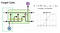Forget Gate

The first step is to decide what information we’re going to throw away from the cell state. This decision is made by a sigmoid layer called the “Forget Gate layer.”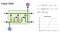Input Gate

The next step is to decide what new information we’re going to store in the cell state. This has two parts. First, a sigmoid layer called the “Input Gate layer” decides which values we’ll update. Next, a tanh layer creates a vector of new candidate values that could be added to the state.

In the next step, we’ll combine these two to create an update to the cell state.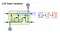Cell State Upates

Then we update the old cell state into the new cell state.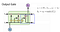Output Gate

Finally, we need to decide what we are going to output. This output will be based on our cell state, but will be a filtered version. First, we run a sigmoid layer which decides what parts of the cell state we’re going to output. Then, we put the cell state through tanh (to push the values to be between -1 and 1) and multiply it by the output of the sigmoid gate, so that we only output the parts we decided to.

## Problem Definition

For our problem definition, we’ll try to solve the problem on the text classification on BBC News articles and its category. Based on the given text as an input, we will predict what would be the category. We have five type of categories: business, entertainment, politics, sport and tech.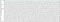BBC News Articles with category

## Import the Libraries

Let’s get started. Firstly, we will import the necessary libraries like TensorFlow, Numpy and CSV.

## Get the Data

We need a data for our model, we will get the data from https://storage.googleapis.com/dataset-uploader/bbc/bbc-text.csv and save it in a /tmp folder with the file name bbc-text.csv.

## Import NTLK Library

We import ntlk library and import the stopwords function. We will set the stopwords for English language. These are the samples for English stopwords: has, hasn’t, and, aren’t, because, each, during.

## Set the Hyper-Parameters

We set the hyper-Parameters that are required to build and train the model.

## Populate List and Remove the Stopwords

We populate the list of articles and labels from the data and also remove the stopwords.

For example, the original text before removing the stopwords is:

And after removing the stopwords, it will become like this:

Let’s print the total number of labels and articles.

print(len(labels))
print(len(articles))

We will get 2225 for labels and 2225 for articles.

## Create Training and Validation Set

Then we need to split them into training set and validation set. We set 80% (training_portion = .8) for training and another 20% for validation.

Let’s print the each one of these:

print(“train_size”, train_size)

print(f”train_articles {len(train_articles)}”)

print(“train_labels”, len(train_labels))

print(“validation_articles”, len(validation_articles))

print(“validation_labels”, len(validation_labels))

and you’ll get:

## Tokenization

We set the tokenization with num_words is vocab_size (5000), and oov_token is ‘<OOV>’ . And we call the method fits_on_texts on train_articles. This method creates the vocabulary index based on word frequency.
For example if we give the text “The cat sat on the mat.”, it will create a dictonary {‘<OOV>’: 1, ‘cat’: 3, ‘mat’: 6, ‘on’: 5, ‘sat’: 4, ‘the’: 2}.

The oov_token is the value ‘<OOV>’ that we put if the word is not listed in the dictionary.

## Convert to Sequences

Machine learning works well with the numbers. After tokenization, we call the method text_to_sequences. It transforms each text in texts to a sequence of integers. Basically, it takes each word in the text and replaces it with its corresponding integer value from the dictionary tokenizer.word_index. If the word is not in the dictionary, it will put the value of 1.
For example, if we give the text “the cat sat on my table”, we will get the sequence: [2, 3, 4, 5, 1, 1]. The last two [1,1] is for the word “my table which is not in the dictionary.

## Sequence Truncation and Padding

Those sequences are not in the same size, we need to make them in same size (concrete shape) when we train them for NLP. We need to use padding and truncate them so all sequences will be in the same size. We use post for padding_type and truncate_type.

Based on the previous sequences, if we pad and truncate them with the maximum length of 10 and padding_type and set truncating_type to post, we will get the below result. See the the four zeros at the end. The length should be 10.

If we set padding_type and truncating_type to pre, we will get the four zeros at the beginning.

We will apply tokenization, convert to sequences and padding/truncating to train_articles and validation_articles.

## Labels

Now let’s take a look at label.

We need to do the same thing here as we did before when we deal with the features, articles. As the model doesn’t understand the words, we need to convert the label into numbers. We do tokenization and convert to sequence as before. When doing the tokenization, we don’t indicate the vocab size and oov_token.

The sequence of the dictionary is important when we do the prediction later.

## Create Model

Now we are ready to create the sequential model. The model architecture consist of the following layers:

• Embedding Layer
The model begins with an embedding layer which turns the input integer indices into the corresponding word vectors. Word embedding is a way to represent a word as a vector. Word embeddings allow the value of the vector’s element to be trained. After training, words with similar meanings often have the similar vectors.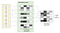A schematic illustration of how an embedding matrix works. Each row of the embedding matrix corresponds to a word in the vocabulary, and each column is an embedding dimension. The values of the embedding matrix’s elements, represented as shades of gray in the diagram, are chosen at random. Source: Deep Learning with JavaScript book.
• Dropout Layer
Add the dropout layer to combat overfitting.
• BiDirectional with LSTM Layer
The BiDirectional layer propagates the input forward and backwards through the LSTM layer and then concatenates the output. This helps the LSTM to learn long range dependencies.
• Dense Layer
This the final layer, Dense layer with softmax activation for the multi class classification.

## Compile the Model

We then compile the model to configure the training process with the loss sparse_categorical_crossentropy since we didn’t one-hot encode the labels. We use Adam optimizer.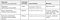Choosing last-layer activation, loss and metrics function. Taken from Deep Learning with JavaScript book.

## Train the Model

Now we are ready to train the model by calling the method fit().

I only use 10 epochs and we can get the “not too bad” accuracy.

We plot the history for accuracy and loss and see if there is overfitting.

## Prediction

Finally, we call the method predict() to perform prediction on the text.

It is correctly predicted as politics.

But not this one. It is incorrectly predicted as politics. Actual label is business.

## More from Ferry Djaja

https://www.linkedin.com/in/ferrydjaja/